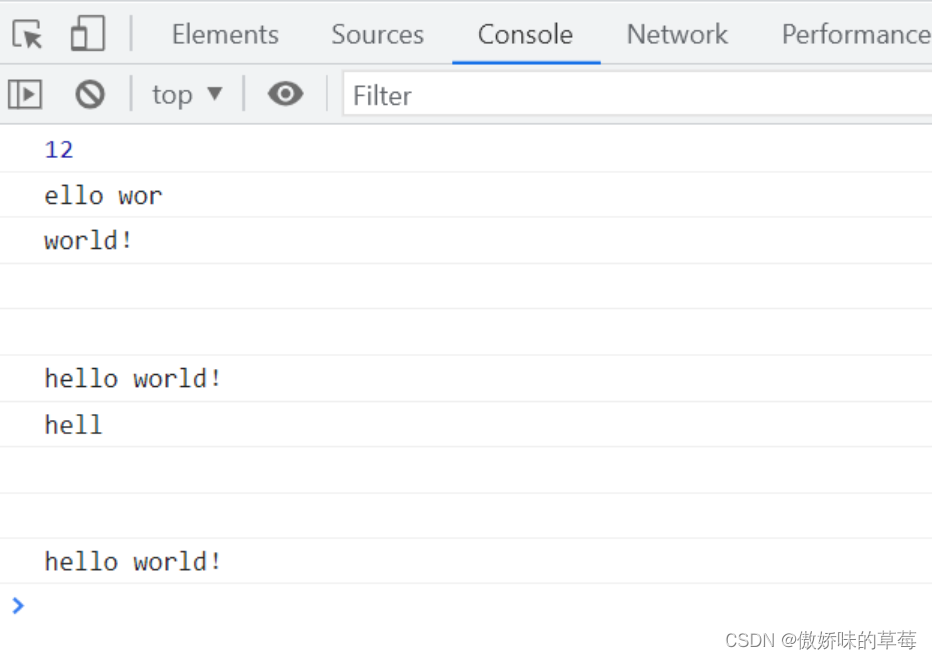﻿ js字符串分割处理的几种方法(6种)_javascript技巧_脚本之家
javascript技巧# js字符串分割处理的几种方法(6种)

## 1、slice(start, end)

```    let test = 'hello world！'
console.log(test.length)

console.log(test.slice(1, 9))
console.log(test.slice(6))
console.log(test.slice(9, 1))
console.log(test.slice(-2))
console.log(test.slice(0, -2))
console.log(test.slice(-4, -2))
console.log(test.slice(-2, 4))
```①第一个参数比第二个参数大，结果返回空字符串

②传入参数是负数，slice()会先做运算 test.length + 负数参数。

## 2、substr(start, length)

```    let test = 'hello world！'
console.log(test.length)

console.log(test.substr(1, 9))
console.log(test.substr(6))
console.log(test.substr(9, 9))
console.log(test.substr(20))
console.log(test.substr(-2))
console.log(test.substr(-8, 4))
console.log(test.substr(-8, 0))
console.log(test.substr(-8, -4))
console.log(test.substr(-20))
```①传入参数超过length返回空字符串

②传入负数，则从字符串的尾部开始算起始位置，-1指最后一个字符，-2指倒数第二个字符；当传入的第一个参数是负数且它的绝对值超过length，这个负数转化为0，当传入的第二个参数是负数，等价于0，截取0个字符，返回空字符串。

## 3、substring(start, stop)

```    let test = 'hello world！'
console.log(test.length)

console.log(test.substring(1, 9))
console.log(test.substring(6))
console.log(test.substring(9, 9))
console.log(test.substring(20))
console.log(test.substring(-2))
console.log(test.substring(-8, 4))
console.log(test.substring(-8, 0))
console.log(test.substring(-8, -4))
console.log(test.substring(-20))
```①第二个参数==第一个参数，返回空字符串

②传入两个参数，不管在第一还是第二位置，都会将小的参数作为第一个参数，较大的作为第二个参数

③任何一个参数为负数或者NaN的时候，自动将其转换为0

④任何一个参数大于length，按照length处理

js字符串截取三姐妹，都不会对原始的字符串进行修改，而是返回新的子集。但是三姐妹各自有各自的个性，面对同一种参数处理的方式都是不一样的。

## 4、split(separator, length)

```//以空格为分隔符输出数组
var str = '123 abc 1 2 3 a b c '
var arr = str.split(' ')
console.log(arr)
``````    var str = '123 abc 1 2 3 a b c'
var arr = str.split(' ', 4)
//第二个参数表示返回数组的最大长度！注意不是原来字符串的，是新输出的数组的
console.log(arr)
```## 5、join(separator)

```    var a = ['I', 'am', 'a', 'girl', '英文名', '是', 'gaby']
var arr = a.join(',')
console.log(arr)
```## 6、splice(start, length, …args)

```//删，第一个参数为第一项位置，第二个参数为要删除几个 0数起
//array.splice(index,num)，返回值为删除内容，array为结果值
var arr = ['a', 'b', 'c', 'd', 'e', 'f']
console.log(arr.splice(0, 4))
console.log(arr)
``````//增，第一个参数（插入位置），第二个参数（0），第三个参数（插入的项）
//array.splice(index,0,insertValue)，返回值为空数组，array值为最终结果值
var arr = ['a', 'b', 'c', 'd', 'e', 'f']
console.log(arr.splice(2, 0, 'insert'))
console.log(arr)
``````//改 第一个参数（起始位置），第二个参数（删除的项数），第三个参数（插入任意数量的项）
//array.splice(index,num,insertValue)，返回值为删除内容，array为结果值
var arr = ['a', 'b', 'c', 'd', 'e', 'f']
console.log(arr.splice(2, 1, 'delete'))
console.log(arr)
```到此这篇关于js字符串分割处理的几种方法(6种)的文章就介绍到这了,更多相关js字符串分割处理内容请搜索脚本之家以前的文章或继续浏览下面的相关文章希望大家以后多多支持脚本之家！